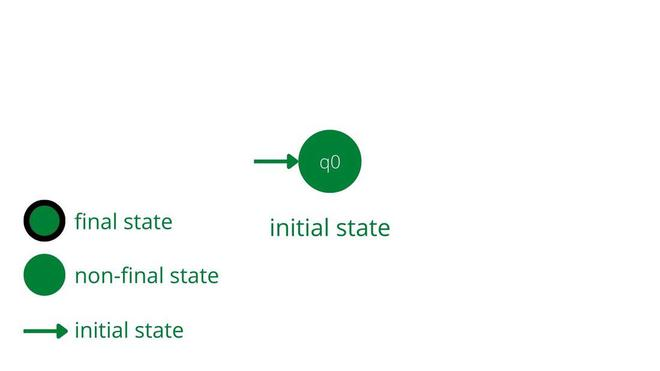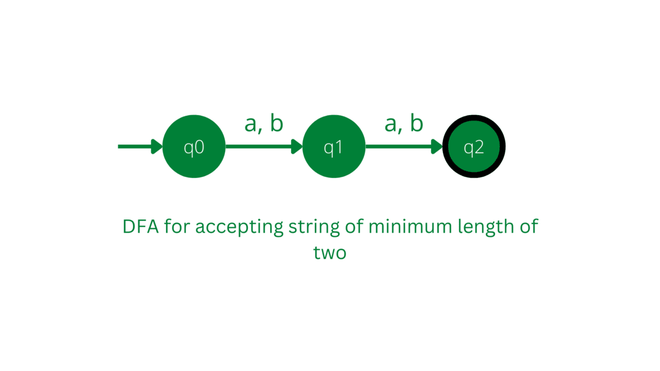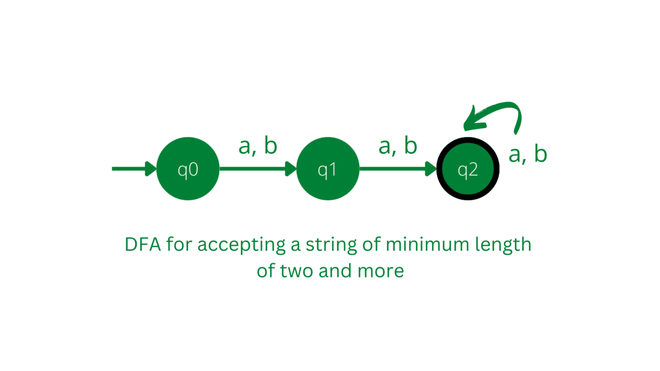GFG App
Open AppBrowser
Continue

# Construct DFA with Σ = {0, 1} and Accept All String of Length at Least 2

Construct a DFA for a language accepting strings of length at least two, over input alphabets Σ = {0,1}. So that means in DFA, language consists of a string of length of at least 2 and can be greater than two. That means if DFA got the string of Length 0 or 1 then it will not accept it. A string of length zero means when the machine doesn’t get any symbol as an input.

### Approach:

• First, try to make the language with the help of string conditions.
• Find the minimum possible string that satisfies the condition of the length of at least two.
• So, the required strings with lengths of 2 and more will be the strings in the given language.

### Concept:

We need a language that will accept a string of length of at least 2 and can be greater than 2. So that means strings with lengths zero and one, will not be part of the language but apart from that, every string can be accepted by a given language. So firstly we will make DFA for the minimum string here of length 2 and then later, we don’t have any restrictions of input alphabets a and b so we will accept everything with any sequence.

Let’s discuss the stepwise construction of DFA:

### Steps for Construction:

Accepting minimum string of length 2: We need a DFA which will accept strings of length two. So for that, we’ll make an initial state for DFA named q0 followed by two states q1 and q2 which will be able to accept a string of length of exactly 2.Initializing the start state and conventions for states

Now see the DFA which will accept the string of length exact two:Accepting string of length at least two: Above DFA don’t have any transition for a and b. So according to the given language, we need a string with a length of two and more than that, so we are done with a minimum string of length 2. For string that has a length greater than 2. We need a self-loop on state q2 for input alphabets a and b. So it can be capable of generating a string of length greater than two.This is the final DFA which is capable of accepting strings of length at least two or lengths of two and more than that.

## C++

 `#include ` `#include `   `class` `LengthAtLeastTwoDFA {` `private``:` `    ``static` `const` `int` `Q0 = 0; ``// initial state` `    ``static` `const` `int` `Q1 = 1; ``// final state` `public``:` `    ``bool` `accept(std::string input) {` `        ``int` `state = Q0;` `        ``for` `(``char` `c : input) {` `            ``switch` `(state) {` `                ``case` `Q0:` `                    ``if` `(c == ``'0'` `|| c == ``'1'``) {` `                        ``state = Q1;` `                    ``} ``else` `{` `                        ``return` `false``; ``// reject input` `                    ``}` `                    ``break``;` `                ``case` `Q1:` `                    ``if` `(c == ``'0'` `|| c == ``'1'``) {` `                        ``state = Q1;` `                    ``} ``else` `{` `                        ``return` `true``; ``// accept input` `                    ``}` `                    ``break``;` `            ``}` `        ``}` `        ``return` `false``; ``// reject input of length 1 or less` `    ``}` `};`   `int` `main() {` `    ``LengthAtLeastTwoDFA dfa;` `    ``std::string input;` `    ``std::cout << ``"Enter input string: "``;` `    ``std::cin >> input;` `    ``if` `(dfa.accept(input)) {` `        ``std::cout << ``"Accepted\n"``;` `    ``} ``else` `{` `        ``std::cout << ``"Rejected\n"``;` `    ``}` `    ``return` `0;` `}`

## Java

 `// DFA that accepts all strings of length at least 2 over the alphabet {0, 1}` `public` `class` `LengthAtLeastTwoDFA {`   `    ``private` `static` `final` `int` `Q0 = ``0``; ``// initial state` `    ``private` `static` `final` `int` `Q1 = ``1``; ``// final state`   `    ``public` `boolean` `accept(String input) {` `        ``int` `state = Q0;` `        ``for` `(``char` `c : input.toCharArray()) {` `            ``switch` `(state) {` `                ``case` `Q0:` `                    ``if` `(c == ``'0'` `|| c == ``'1'``) {` `                        ``state = Q1;` `                    ``} ``else` `{` `                        ``return` `false``; ``// reject input` `                    ``}` `                    ``break``;` `                ``case` `Q1:` `                    ``if` `(c == ``'0'` `|| c == ``'1'``) {` `                        ``state = Q1;` `                    ``} ``else` `{` `                        ``return` `true``; ``// accept input` `                    ``}` `                    ``break``;` `            ``}` `        ``}` `        ``return` `false``; ``// reject input of length 1 or less` `    ``}` `}`

## Python3

 `class` `LengthAtLeastTwoDFA:` `    ``Q0 ``=` `0`  `# initial state` `    ``Q1 ``=` `1`  `# final state`   `    ``def` `accept(``self``, input_str):` `        ``state ``=` `LengthAtLeastTwoDFA.Q0` `        ``for` `c ``in` `input_str:` `            ``if` `state ``=``=` `LengthAtLeastTwoDFA.Q0:` `                ``if` `c ``=``=` `'0'` `or` `c ``=``=` `'1'``:` `                    ``state ``=` `LengthAtLeastTwoDFA.Q1` `                ``else``:` `                    ``return` `False`  `# reject input` `            ``elif` `state ``=``=` `LengthAtLeastTwoDFA.Q1:` `                ``if` `c ``=``=` `'0'` `or` `c ``=``=` `'1'``:` `                    ``state ``=` `LengthAtLeastTwoDFA.Q1` `                ``else``:` `                    ``return` `True`  `# accept input` `        ``return` `False`  `# reject input of length 1 or less`     `if` `__name__ ``=``=` `'__main__'``:` `    ``dfa ``=` `LengthAtLeastTwoDFA()` `    ``input_str ``=` `input``(``"Enter input string: "``)` `    ``if` `dfa.accept(input_str):` `        ``print``(``"Accepted"``)` `    ``else``:` `        ``print``(``"Rejected"``)`

## C#

 `using` `System;`   `public` `class` `LengthAtLeastTwoDFA` `{` `    ``private` `const` `int` `Q0 = 0; ``// initial state` `    ``private` `const` `int` `Q1 = 1; ``// final state`   `    ``public` `bool` `Accept(``string` `input)` `    ``{` `        ``int` `state = Q0;` `        ``foreach` `(``char` `c ``in` `input)` `        ``{` `            ``switch` `(state)` `            ``{` `                ``case` `Q0:` `                    ``if` `(c == ``'0'` `|| c == ``'1'``)` `                    ``{` `                        ``state = Q1;` `                    ``}` `                    ``else` `                    ``{` `                        ``return` `false``; ``// reject input` `                    ``}` `                    ``break``;` `                ``case` `Q1:` `                    ``if` `(c == ``'0'` `|| c == ``'1'``)` `                    ``{` `                        ``state = Q1;` `                    ``}` `                    ``else` `                    ``{` `                        ``return` `true``; ``// accept input` `                    ``}` `                    ``break``;` `            ``}` `        ``}` `        ``return` `false``; ``// reject input of length 1 or less` `    ``}` `}`

## Javascript

 `class LengthAtLeastTwoDFA {` `  ``static Q0 = 0; ``// initial state` `  ``static Q1 = 1; ``// final state`   `  ``accept(inputStr) {` `    ``let state = LengthAtLeastTwoDFA.Q0;` `    ``for` `(const c of inputStr) {` `      ``if` `(state === LengthAtLeastTwoDFA.Q0) {` `        ``if` `(c === ``'0'` `|| c === ``'1'``) {` `          ``state = LengthAtLeastTwoDFA.Q1;` `        ``} ``else` `{` `          ``return` `false``; ``// reject input` `        ``}` `      ``} ``else` `if` `(state === LengthAtLeastTwoDFA.Q1) {` `        ``if` `(c === ``'0'` `|| c === ``'1'``) {` `          ``state = LengthAtLeastTwoDFA.Q1;` `        ``} ``else` `{` `          ``return` `true``; ``// accept input` `        ``}` `      ``}` `    ``}` `    ``return` `false``; ``// reject input of length 1 or less` `  ``}` `}`   `const dfa = ``new` `LengthAtLeastTwoDFA();` `const inputStr = prompt(``"Enter input string: "``);` `if` `(dfa.accept(inputStr)) {` `  ``console.log(``"Accepted"``);` `} ``else` `{` `  ``console.log(``"Rejected"``);` `}`

My Personal Notes arrow_drop_up